## Cohesion and Adhesion in Liquids: Surface Tension and Capillary Action

### Learning Objectives

By the end of this section, you will be able to:

• Understand cohesive and adhesive forces.
• Define surface tension.
• Understand capillary action.

## Cohesion and Adhesion in LiquidsFigure 1. The soap bubbles in this photograph are caused by cohesive forces among molecules in liquids. (credit: Steve Ford Elliott)

Children blow soap bubbles and play in the spray of a sprinkler on a hot summer day. (See Figure 1.) An underwater spider keeps his air supply in a shiny bubble he carries wrapped around him. A technician draws blood into a small-diameter tube just by touching it to a drop on a pricked finger. A premature infant struggles to inflate her lungs. What is the common thread? All these activities are dominated by the attractive forces between atoms and molecules in liquids—both within a liquid and between the liquid and its surroundings.

Attractive forces between molecules of the same type are called cohesive forces. Liquids can, for example, be held in open containers because cohesive forces hold the molecules together. Attractive forces between molecules of different types are called adhesive forces. Such forces cause liquid drops to cling to window panes, for example. In this section we examine effects directly attributable to cohesive and adhesive forces in liquids.

### Cohesive Forces

Attractive forces between molecules of the same type are called cohesive forces.

Attractive forces between molecules of different types are called adhesive forces.

## Surface Tension

Cohesive forces between molecules cause the surface of a liquid to contract to the smallest possible surface area. This general effect is called surface tension. Molecules on the surface are pulled inward by cohesive forces, reducing the surface area. Molecules inside the liquid experience zero net force, since they have neighbors on all sides.

### Surface Tension

Cohesive forces between molecules cause the surface of a liquid to contract to the smallest possible surface area. This general effect is called surface tension.

### Making Connections: Surface Tension

Forces between atoms and molecules underlie the macroscopic effect called surface tension. These attractive forces pull the molecules closer together and tend to minimize the surface area. This is another example of a submicroscopic explanation for a macroscopic phenomenon.

The model of a liquid surface acting like a stretched elastic sheet can effectively explain surface tension effects. For example, some insects can walk on water (as opposed to floating in it) as we would walk on a trampoline—they dent the surface as shown in Figure 2(a). Figure 2(b) shows another example, where a needle rests on a water surface. The iron needle cannot, and does not, float, because its density is greater than that of water. Rather, its weight is supported by forces in the stretched surface that try to make the surface smaller or flatter. If the needle were placed point down on the surface, its weight acting on a smaller area would break the surface, and it would sink.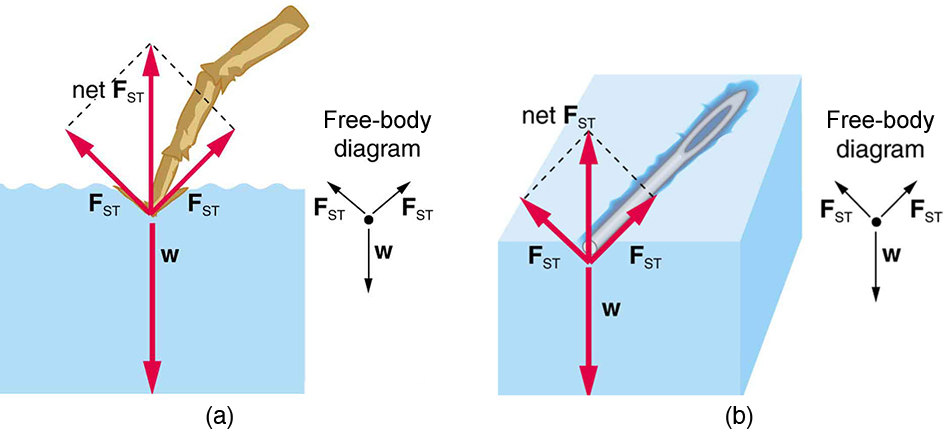Figure 2. Surface tension supporting the weight of an insect and an iron needle, both of which rest on the surface without penetrating it. They are not floating; rather, they are supported by the surface of the liquid. (a) An insect leg dents the water surface. FST is a restoring force (surface tension) parallel to the surface. (b) An iron needle similarly dents a water surface until the restoring force (surface tension) grows to equal its weight.

Surface tension is proportional to the strength of the cohesive force, which varies with the type of liquid. Surface tension γ is defined to be the force F per unit length L exerted by a stretched liquid membrane:

$\gamma =\frac{F}{L}\\$.

Table 1. Surface Tension of Some Liquids
Liquid Surface tension γ(N/m)
Water at 0ºC 0.0756
Water at 20ºC 0.0728
Water at 100ºC 0.0589
Soapy water (typical) 0.0370
Ethyl alcohol 0.0223
Glycerin 0.0631
Mercury 0.465
Olive oil 0.032
Tissue fluids (typical) 0.050
Blood, whole at 37ºC 0.058
Blood plasma at 37ºC 0.073
Gold at 1070ºC 1.000
Oxygen at −193ºC 0.0157
Helium at −269ºC 0.00012

Table 1 above lists values of γ for some liquids. For the insect of Figure 2(a), its weight w is supported by the upward components of the surface tension force: γL sin θ, where L is the circumference of the insect’s foot in contact with the water. Figure 3 shows one way to measure surface tension. The liquid film exerts a force on the movable wire in an attempt to reduce its surface area. The magnitude of this force depends on the surface tension of the liquid and can be measured accurately. Surface tension is the reason why liquids form bubbles and droplets. The inward surface tension force causes bubbles to be approximately spherical and raises the pressure of the gas trapped inside relative to atmospheric pressure outside. It can be shown that the gauge pressure P inside a spherical bubble is given by

$P=\frac{4\gamma }{r}\\$,

where r is the radius of the bubble.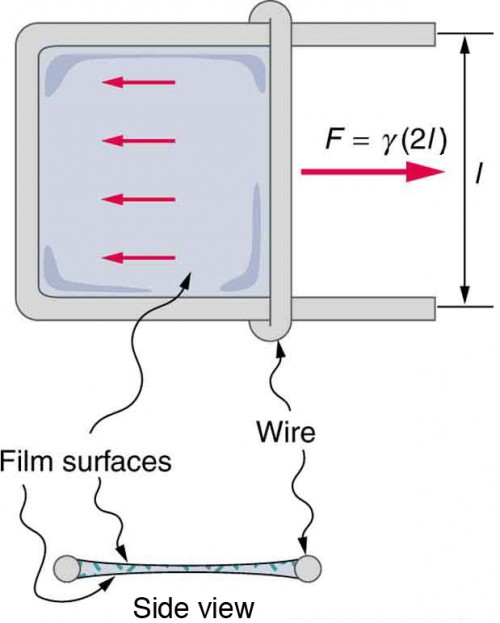Figure 3. Sliding wire device used for measuring surface tension; the device exerts a force to reduce the film’s surface area. The force needed to hold the wire in place is F = γL = γ(2l), since there are two liquid surfaces attached to the wire. This force remains nearly constant as the film is stretched, until the film approaches its breaking point.

Thus the pressure inside a bubble is greatest when the bubble is the smallest. Another bit of evidence for this is illustrated in Figure 4. When air is allowed to flow between two balloons of unequal size, the smaller balloon tends to collapse, filling the larger balloon.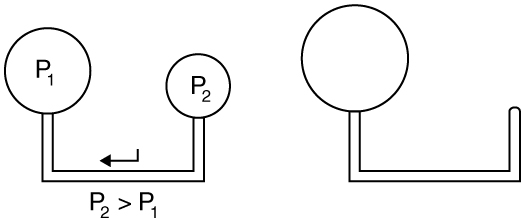Figure 4. With the valve closed, two balloons of different sizes are attached to each end of a tube. Upon opening the valve, the smaller balloon decreases in size with the air moving to fill the larger balloon. The pressure in a spherical balloon is inversely proportional to its radius, so that the smaller balloon has a greater internal pressure than the larger balloon, resulting in this flow.

### Example 1. Surface Tension: Pressure Inside a Bubble

Calculate the gauge pressure inside a soap bubble 2.00 × 10-4 m in radius using the surface tension for soapy water in Table 1. Convert this pressure to mm Hg.

#### Strategy

The radius is given and the surface tension can be found in Table 1, and so P can be found directly from the equation $P=\frac{4\gamma }{r}\\$.

#### Solution

Substituting r and γ into the equation $P=\frac{4\gamma }{r}\\$, we obtain

$P=\frac{4\gamma }{r}=\frac{4\left(0.037\text{ N/m}\right)}{2\text{.}\text{00}\times {\text{10}}^{-4}\text{m}}=\text{740}{\text{ N/m}}^{2}=\text{740}\text{ Pa}\\$.

We use a conversion factor to get this into units of mm Hg:

$P=\left(\text{740}{\text{ N/m}}^{2}\right)\frac{1.00 \text{ mm Hg}}{\text{133}{\text{ N/m}}^{2}}=5.56\text{ mm Hg}\\$.

#### Discussion

Note that if a hole were to be made in the bubble, the air would be forced out, the bubble would decrease in radius, and the pressure inside would increase to atmospheric pressure (760 mm Hg).

Our lungs contain hundreds of millions of mucus-lined sacs called alveoli, which are very similar in size, and about 0.1 mm in diameter. (See Figure 5.) You can exhale without muscle action by allowing surface tension to contract these sacs. Medical patients whose breathing is aided by a positive pressure respirator have air blown into the lungs, but are generally allowed to exhale on their own. Even if there is paralysis, surface tension in the alveoli will expel air from the lungs. Since pressure increases as the radii of the alveoli decrease, an occasional deep cleansing breath is needed to fully reinflate the alveoli. Respirators are programmed to do this and we find it natural, as do our companion dogs and cats, to take a cleansing breath before settling into a nap.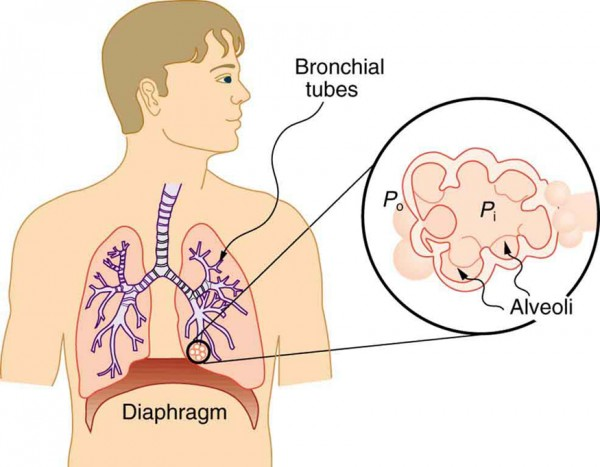Figure 5. Bronchial tubes in the lungs branch into ever-smaller structures, finally ending in alveoli. The alveoli act like tiny bubbles. The surface tension of their mucous lining aids in exhalation and can prevent inhalation if too great.

The tension in the walls of the alveoli results from the membrane tissue and a liquid on the walls of the alveoli containing a long lipoprotein that acts as a surfactant (a surface-tension reducing substance). The need for the surfactant results from the tendency of small alveoli to collapse and the air to fill into the larger alveoli making them even larger (as demonstrated in Figure 4). During inhalation, the lipoprotein molecules are pulled apart and the wall tension increases as the radius increases (increased surface tension). During exhalation, the molecules slide back together and the surface tension decreases, helping to prevent a collapse of the alveoli. The surfactant therefore serves to change the wall tension so that small alveoli don’t collapse and large alveoli are prevented from expanding too much. This tension change is a unique property of these surfactants, and is not shared by detergents (which simply lower surface tension). (See Figure 6.)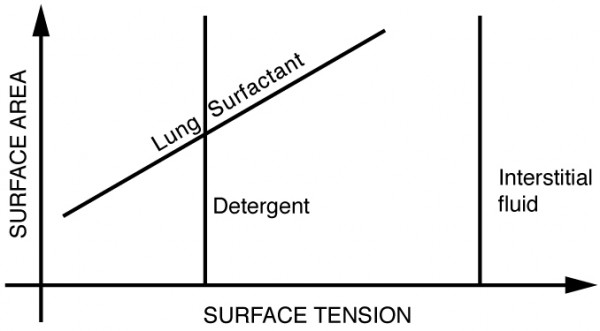Figure 6. Surface tension as a function of surface area. The surface tension for lung surfactant decreases with decreasing area. This ensures that small alveoli don’t collapse and large alveoli are not able to over expand.

If water gets into the lungs, the surface tension is too great and you cannot inhale. This is a severe problem in resuscitating drowning victims. A similar problem occurs in newborn infants who are born without this surfactant—their lungs are very difficult to inflate. This condition is known as hyaline membrane disease and is a leading cause of death for infants, particularly in premature births. Some success has been achieved in treating hyaline membrane disease by spraying a surfactant into the infant’s breathing passages. Emphysema produces the opposite problem with alveoli. Alveolar walls of emphysema victims deteriorate, and the sacs combine to form larger sacs. Because pressure produced by surface tension decreases with increasing radius, these larger sacs produce smaller pressure, reducing the ability of emphysema victims to exhale. A common test for emphysema is to measure the pressure and volume of air that can be exhaled.

### Making Connections: Take-Home Investigation

(1) Try floating a sewing needle on water. In order for this activity to work, the needle needs to be very clean as even the oil from your fingers can be sufficient to affect the surface properties of the needle. (2) Place the bristles of a paint brush into water. Pull the brush out and notice that for a short while, the bristles will stick together. The surface tension of the water surrounding the bristles is sufficient to hold the bristles together. As the bristles dry out, the surface tension effect dissipates. (3) Place a loop of thread on the surface of still water in such a way that all of the thread is in contact with the water. Note the shape of the loop. Now place a drop of detergent into the middle of the loop. What happens to the shape of the loop? Why? (4) Sprinkle pepper onto the surface of water. Add a drop of detergent. What happens? Why? (5) Float two matches parallel to each other and add a drop of detergent between them. What happens? Note: For each new experiment, the water needs to be replaced and the bowl washed to free it of any residual detergent.

## Adhesion and Capillary Action

Why is it that water beads up on a waxed car but does not on bare paint? The answer is that the adhesive forces between water and wax are much smaller than those between water and paint. Competition between the forces of adhesion and cohesion are important in the macroscopic behavior of liquids. An important factor in studying the roles of these two forces is the angle θ between the tangent to the liquid surface and the surface. (See Figure 7.) The contact angle θ is directly related to the relative strength of the cohesive and adhesive forces. The larger the strength of the cohesive force relative to the adhesive force, the larger θ is, and the more the liquid tends to form a droplet. The smaller θ is, the smaller the relative strength, so that the adhesive force is able to flatten the drop. Table 2 lists contact angles for several combinations of liquids and solids.

### Contact Angle

The angle θ between the tangent to the liquid surface and the surface is called the contact angle.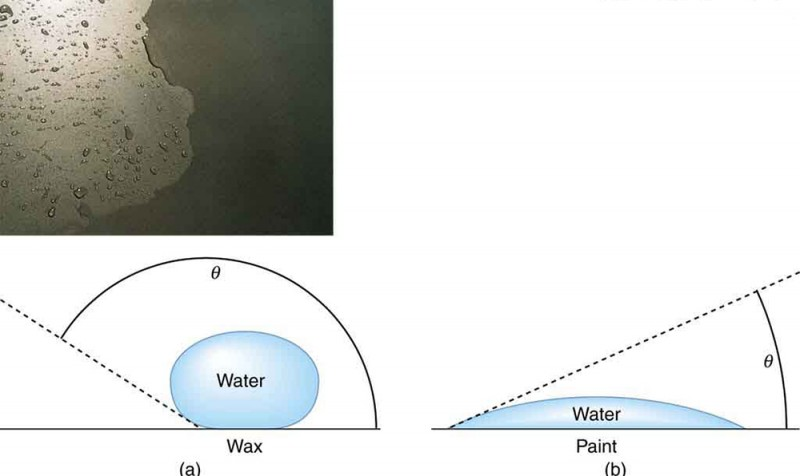Figure 7. In the photograph, water beads on the waxed car paint and flattens on the unwaxed paint. (a) Water forms beads on the waxed surface because the cohesive forces responsible for surface tension are larger than the adhesive forces, which tend to flatten the drop. (b) Water beads on bare paint are flattened considerably because the adhesive forces between water and paint are strong, overcoming surface tension. The contact angle θ is directly related to the relative strengths of the cohesive and adhesive forces. The larger θ is, the larger the ratio of cohesive to adhesive forces. (credit: P. P. Urone)

One important phenomenon related to the relative strength of cohesive and adhesive forces is capillary action—the tendency of a fluid to be raised or suppressed in a narrow tube, or capillary tube. This action causes blood to be drawn into a small-diameter tube when the tube touches a drop.

### Capillary Action

The tendency of a fluid to be raised or suppressed in a narrow tube, or capillary tube, is called capillary action.

If a capillary tube is placed vertically into a liquid, as shown in Figure 8, capillary action will raise or suppress the liquid inside the tube depending on the combination of substances. The actual effect depends on the relative strength of the cohesive and adhesive forces and, thus, the contact angle θ given in the table. If θ is less than 90º, then the fluid will be raised; if θ is greater than 90º, it will be suppressed. Mercury, for example, has a very large surface tension and a large contact angle with glass. When placed in a tube, the surface of a column of mercury curves downward, somewhat like a drop. The curved surface of a fluid in a tube is called a meniscus. The tendency of surface tension is always to reduce the surface area. Surface tension thus flattens the curved liquid surface in a capillary tube. This results in a downward force in mercury and an upward force in water, as seen in Figure 8.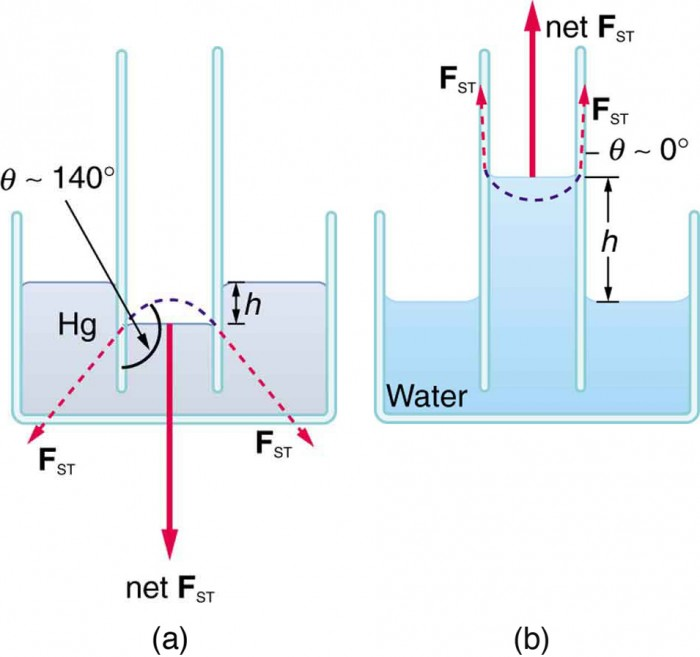Figure 8. (a) Mercury is suppressed in a glass tube because its contact angle is greater than 90º. Surface tension exerts a downward force as it flattens the mercury, suppressing it in the tube. The dashed line shows the shape the mercury surface would have without the flattening effect of surface tension. (b) Water is raised in a glass tube because its contact angle is nearly 0º. Surface tension therefore exerts an upward force when it flattens the surface to reduce its area.

Table 2. Contact Angles of Some Substances
Interface Contact angle Θ
Mercury–glass 140º
Water–glass
Water–paraffin 107º
Water–silver 90º
Organic liquids (most)–glass
Ethyl alcohol–glass
Kerosene–glass 26º

Capillary action can move liquids horizontally over very large distances, but the height to which it can raise or suppress a liquid in a tube is limited by its weight. It can be shown that this height h is given by

$h=\frac{2\gamma \cos\theta}{\rho{gr}}\\$.

If we look at the different factors in this expression, we might see how it makes good sense. The height is directly proportional to the surface tension γ, which is its direct cause. Furthermore, the height is inversely proportional to tube radius—the smaller the radius r, the higher the fluid can be raised, since a smaller tube holds less mass. The height is also inversely proportional to fluid density ρ, since a larger density means a greater mass in the same volume. (See Figure 9.)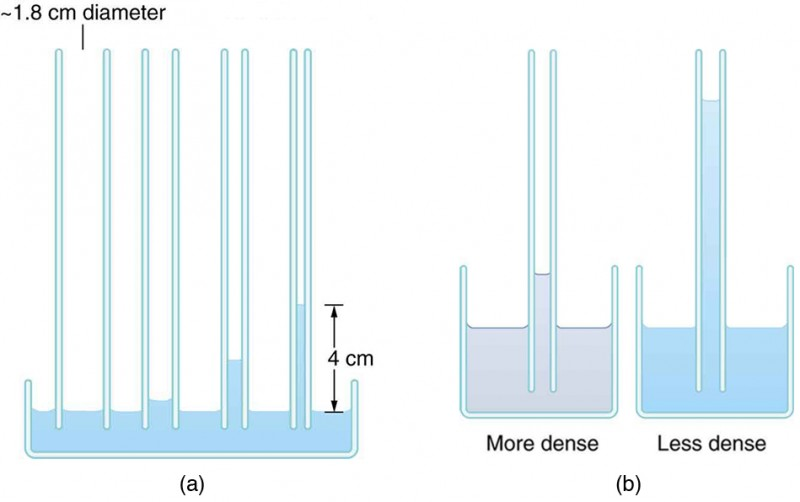Figure 9. (a) Capillary action depends on the radius of a tube. The smaller the tube, the greater the height reached. The height is negligible for large-radius tubes. (b) A denser fluid in the same tube rises to a smaller height, all other factors being the same.

### Example 2. Calculating Radius of a Capillary Tube: Capillary Action: Tree Sap

Can capillary action be solely responsible for sap rising in trees? To answer this question, calculate the radius of a capillary tube that would raise sap 100 m to the top of a giant redwood, assuming that sap’s density is 1050 kg/m3, its contact angle is zero, and its surface tension is the same as that of water at 20.0º C.

#### Strategy

The height to which a liquid will rise as a result of capillary action is given by $h=\frac{2\gamma \cos\theta}{\rho\text{gr}}\\$, and every quantity is known except for r.

#### Solution

Solving for r and substituting known values produces

$\begin{array}{lll}r& =& \frac{2\gamma\cos\theta}{\rho \text{gh}}=\frac{2\left(\text{0.0728 N/m}\right)\cos\left(0º\right)}{\left({1050}{\text{ kg/m}}^{3}\right)\left(9\text{.}\text{80}{\text{ m/s}}^{2}\right)\left(\text{100 m}\right)}\\ & =& 1.41\times {\text{10}}^{-7}\text{m.}\end{array}\\$

#### Discussion

This result is unreasonable. Sap in trees moves through the xylem, which forms tubes with radii as small as 2.5 × 10-5 m. This value is about 180 times as large as the radius found necessary here to raise sap 100 m. This means that capillary action alone cannot be solely responsible for sap getting to the tops of trees.

How does sap get to the tops of tall trees? (Recall that a column of water can only rise to a height of 10 m when there is a vacuum at the top—see Example 3 from Variation of Pressure with Depth in a Fluid.) The question has not been completely resolved, but it appears that it is pulled up like a chain held together by cohesive forces. As each molecule of sap enters a leaf and evaporates (a process called transpiration), the entire chain is pulled up a notch. So a negative pressure created by water evaporation must be present to pull the sap up through the xylem vessels. In most situations, fluids can push but can exert only negligible pull, because the cohesive forces seem to be too small to hold the molecules tightly together. But in this case, the cohesive force of water molecules provides a very strong pull. Figure 10 shows one device for studying negative pressure. Some experiments have demonstrated that negative pressures sufficient to pull sap to the tops of the tallest trees can be achieved.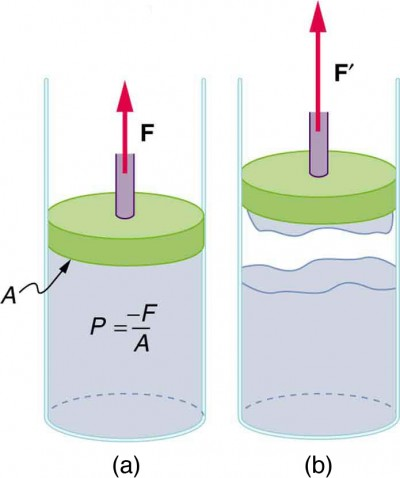Figure 10. (a) When the piston is raised, it stretches the liquid slightly, putting it under tension and creating a negative absolute pressure P = −F/A. (b) The liquid eventually separates, giving an experimental limit to negative pressure in this liquid.

## Section Summary

• Attractive forces between molecules of the same type are called cohesive forces.
• Attractive forces between molecules of different types are called adhesive forces.
• Cohesive forces between molecules cause the surface of a liquid to contract to the smallest possible surface area. This general effect is called surface tension.
• Capillary action is the tendency of a fluid to be raised or suppressed in a narrow tube, or capillary tube which is due to the relative strength of cohesive and adhesive forces.

### Conceptual Questions

1. The density of oil is less than that of water, yet a loaded oil tanker sits lower in the water than an empty one. Why?

2. Is surface tension due to cohesive or adhesive forces, or both?

3. Is capillary action due to cohesive or adhesive forces, or both?

4. Birds such as ducks, geese, and swans have greater densities than water, yet they are able to sit on its surface. Explain this ability, noting that water does not wet their feathers and that they cannot sit on soapy water.

5. Water beads up on an oily sunbather, but not on her neighbor, whose skin is not oiled. Explain in terms of cohesive and adhesive forces.

6. Could capillary action be used to move fluids in a “weightless” environment, such as in an orbiting space probe?

7. What effect does capillary action have on the reading of a manometer with uniform diameter? Explain your answer.

8. Pressure between the inside chest wall and the outside of the lungs normally remains negative. Explain how pressure inside the lungs can become positive (to cause exhalation) without muscle action.

### Problems & Exercises

1. What is the pressure inside an alveolus having a radius of 2.50 × 10-4 if the surface tension of the fluid-lined wall is the same as for soapy water? You may assume the pressure is the same as that created by a spherical bubble.

2. (a) The pressure inside an alveolus with a 2.00 × 10-4 -m radius is 1.40 × 103, due to its fluid-lined walls. Assuming the alveolus acts like a spherical bubble, what is the surface tension of the fluid? (b) Identify the likely fluid. (You may need to extrapolate between values in Table 1.)

3. What is the gauge pressure in millimeters of mercury inside a soap bubble 0.100 m in diameter?

4. Calculate the force on the slide wire in Figure 3 (shown again below) if it is 3.50 cm long and the fluid is ethyl alcohol.Figure 3. Sliding wire device used for measuring surface tension; the device exerts a force to reduce the film’s surface area. The force needed to hold the wire in place is F = γL = γ(2l), since there are two liquid surfaces attached to the wire. This force remains nearly constant as the film is stretched, until the film approaches its breaking point.

5. Figure 9(a) (shown again below) shows the effect of tube radius on the height to which capillary action can raise a fluid. (a) Calculate the height h for water in a glass tube with a radius of 0.900 cm—a rather large tube like the one on the left. (b) What is the radius of the glass tube on the right if it raises water to 4.00 cm?Figure 9. (a) Capillary action depends on the radius of a tube. The smaller the tube, the greater the height reached. The height is negligible for large-radius tubes. (b) A denser fluid in the same tube rises to a smaller height, all other factors being the same.

6. We stated in Example 2 above that a xylem tube is of radius 2.50 × 10-5 m. Verify that such a tube raises sap less than a meter by finding h for it, making the same assumptions that sap’s density is 1050 kg/m3, its contact angle is zero, and its surface tension is the same as that of water at 20.0º C.

7. What fluid is in the device shown in Figure 3 (shown again below) if the force is 3.16 × 10-3 and the length of the wire is 2.50 cm? Calculate the surface tension γ and find a likely match from Table 1 (above).Figure 3. Sliding wire device used for measuring surface tension; the device exerts a force to reduce the film’s surface area. The force needed to hold the wire in place is F = γL = γ(2l), since there are two liquid surfaces attached to the wire. This force remains nearly constant as the film is stretched, until the film approaches its breaking point.

8. If the gauge pressure inside a rubber balloon with a 10.0-cm radius is 1.50 cm of water, what is the effective surface tension of the balloon?

9. Calculate the gauge pressures inside 2.00-cm-radius bubbles of water, alcohol, and soapy water. Which liquid forms the most stable bubbles, neglecting any effects of evaporation?

10. Suppose water is raised by capillary action to a height of 5.00 cm in a glass tube. (a) To what height will it be raised in a paraffin tube of the same radius? (b) In a silver tube of the same radius?

11. Calculate the contact angle θ for olive oil if capillary action raises it to a height of 7.07 cm in a glass tube with a radius of 0.100 mm. Is this value consistent with that for most organic liquids?

12. When two soap bubbles touch, the larger is inflated by the smaller until they form a single bubble. (a) What is the gauge pressure inside a soap bubble with a 1.50-cm radius? (b) Inside a 4.00-cm-radius soap bubble? (c) Inside the single bubble they form if no air is lost when they touch?

13. Calculate the ratio of the heights to which water and mercury are raised by capillary action in the same glass tube.

14. What is the ratio of heights to which ethyl alcohol and water are raised by capillary action in the same glass tube?

## Glossary

the attractive forces between molecules of different types
capillary action:
the tendency of a fluid to be raised or lowered in a narrow tube
cohesive forces:
the attractive forces between molecules of the same type
contact angle:
the angle θ between the tangent to the liquid surface and the surface
surface tension:
the cohesive forces between molecules which cause the surface of a liquid to contract to the smallest possible surface area

### Selected Solutions to Problems & Exercises

1. 592 N/m2

3. 2.23 × 10-2 mm Hg

5. (a) 1.65 × 10-3 m (b) 3.71 × 10-4 m

7. 6.32 × 10-2 N/m. Based on the values in table, the fluid is probably glycerin.

9. $\begin{array}{lll}{P}_{\text{w}}& =& \text{14}\text{.}6{\text{ N/m}}^{2},\\ {P}_{\text{a}}& =& \text{4.46}{\text{ N/m}}^{2},\\ {P}_{\text{sw}}& =& \text{7.40}{\text{ N/m}}^{2}\text{.}\end{array}\\$

Alcohol forms the most stable bubble, since the absolute pressure inside is closest to atmospheric pressure.

11. 5.1º. This is near the value of θ=0º for most organic liquids.

13. -2.78. The ratio is negative because water is raised whereas mercury is lowered.

1. At 20ºC unless otherwise stated.